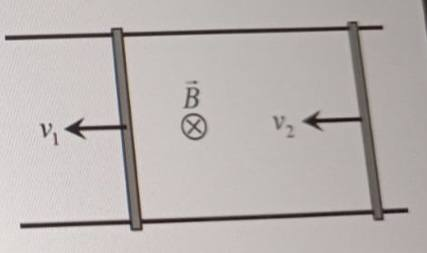# Two metallic bars moving on rails enclosing a vertical B-field

Guillem_dlc
Homework Statement:
Two metallic bars of the same length move on two very long rails, also conductive, with velocities ##v_1## and ##v_2## within a constant magnetic field perpendicular to the plane of the paper and directed inward. We can affirm that:

a) If ##v_1=v_2##, no current will flow through the circuit.
b) If ##v_1>v_2##, a clockwise current will flow through the circuit.
c) If ##v_1<v_2##, a counterclockwise current will flow through the circuit.
d) As the field does not vary with time, the circuit will not flow current in any case.
Relevant Equations:I know the correct answer is a), but I have no idea how to approach the exercise. If you could give me any hints/ideas I would appreciate it.

Homework Helper
Gold Member
2022 Award
What about using the Lorentz Force Law?

Gold Member
OK, so you've mentioned Faraday's Law, what does it say about induced voltages? What would that depend on? You might also consider Lenz's Law which is related.

The link from current to voltage here is that it will require some voltage to cause the current to flow. You can assume a very small but non-zero resistance in the bars and rails.

Homework Helper
Gold Member
I know the correct answer is a), but I have no idea how to approach the exercise. If you could give me any hints/ideas I would appreciate it.
Do you understand the concept of motional emf when a single bar is moving through a magnetic field?
If "no" here is a brief reminder.
If "yes", is your problem not being sure how to apply this idea to two bars electrically connected through the rails?

•Steve4Physics, Delta2 and Lnewqban
Guillem_dlc
I think I got the exercise:
$$\phi_m=\int_S B\, ds=BS \rightarrow d\phi_m=B\, ds$$
$$\varepsilon_{\textrm{ind}}=-\dfrac{d\phi_m}{dt}=-B\dfrac{ds}{dt}$$
If ##v_1=v_2##, then ##dS## does not change ##\rightarrow d\phi_m=0\rightarrow \varepsilon_{\textrm{ind}}=0\rightarrow \boxed{I_{\textrm{ind}}=0}##

Homework Helper
Gold Member
2022 Award
I think I got the exercise:
$$\phi_m=\int_S B\, ds=BS \rightarrow d\phi_m=B\, ds$$
$$\varepsilon_{\textrm{ind}}=-\dfrac{d\phi_m}{dt}=-B\dfrac{ds}{dt}$$
If ##v_1=v_2##, then ##dS## does not change ##\rightarrow d\phi_m=0\rightarrow \varepsilon_{\textrm{ind}}=0\rightarrow \boxed{I_{\textrm{ind}}=0}##
Is this multiple choice (with one correct answer) or is it possible that there is more than one correct answer?

If the former, I think we can establish a) by logic and symmetry alone. E.g. d) cannot be correct without a) also being correct, so that would rule out d) as being the only correct answer.

Homework Helper
Gold Member
Since OP's question has been answered, I have a question related to this. Suppose that at ##t=0##, ##v_1=0## and ##v_2=v_0## and the initial separation is ##d##. The bars may have different masses.

Question: Without writing any differential equations, is a collision always possible, never possible or "it depends"? Note that the question essentially asks, given the picture in post #1, what can we say about what happens next?

My guess is that it depends. I think it is true that the linear momentum of the two-bar system is conserved because the current is the same in both bars therefore the ##I\mathbf{L}\times \mathbf{B}## external forces are equal and opposite whether in attraction or repulsion. Obviously, the bar-to-bar repulsion forces are also equal and opposite if one choses not to ignore them.

The condition for no collision is that the relative velocity become zero before the distance between bars is reduced to zero. Then the bars will be moving with the CoM velocity ##V_{cm}=\frac{m_2}{m_1+m_2}v_0.## just like an inelastic collision except that the mechanical energy has gone into Ohmic losses.

What if there is a collision? Let's assume that the collision conserves mechanical energy. Then the relative velocity will be the same before and after the but the bars will be separating while the magnetic forces on each will be in attraction. More energy will go to Ohmic losses until the relative velocity goes to zero.

In short, this seems to be the Faraday's law equivalent of a perfectly inelastic collision.

Guillem_dlc
It's multiple choice with one correct answer.

Homework Helper
Gold Member
2022 Award
It's multiple choice with one correct answer.
If d) implies a), then it can't be d). And it looks to me that b) and c) are either both correct or both wrong. So, it must be a).

•Delta2
Gold Member
LOL, y'all have gotten too good at multiple choice tests! They ask an EM question but the answer is simple logic.

Anyway, the shortcut answer for me is that the equations can be interpreted as saying "voltage will be induced to cause current to flow that resists any change in B, the flux density." So, if the area increases, current will flow in the polarity and magnitude to add flux to maintain B constant. That plus the right hand polarity rule is the quick solution.

So, just like capacitors make current flow to resist a voltage change, inductors make a voltage to resist a flux density change.

•PhDeezNutz and PeroK
Anyway, for whatever it's worth, I solved the diff eqs under the initial conditions that ##v_2(0)=v_0## and ##v_1(0)=0## and I got $$v_1=\frac{m_2~v_0}{m_1+m_2}\left(1-e^{-\alpha t}\right)~;~~v_2=v_0-\frac{m_1~v_0}{m_1+m_2}\left(1-e^{-\alpha t}\right)~~~\left[\alpha\equiv \frac{(m_1+m_2)}{m_1m_2}\frac{B^2L^2}{2R}\right].$$I assumed that the bars have equal resistance ##R## but different masses. The relative velocity is, as one might expect, $$v_{\text{rel}}=v_2-v_1=v_0~e^{-\alpha~t}.$$On edit: That answers my question. Since the relative velocity goes to zero asymptotically, there will always be a collision if the masses are moving in the same direction initially.